# Constant, Changing, and Average Velocity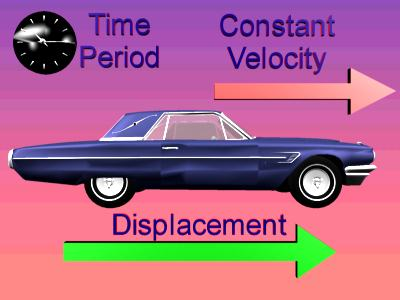In this picture we consider that the car is moving through equal displacements per equal time intervals, and this is what is meant by a constant velocity. Note that the constant velocity would be aimed in the same direction as the equal displacements.

To say that an object has constant velocity means that it is moving along a straight line, and as every second of time goes by, the object travels through the same number of meters. Or we could say that it travels equal displacements per equal time intervals. For example:

• If an automobile was moving down a straight road....
• And in the first second it moved 20 meters....
• And in the next second it moved 20 meters again....
• And in each of the following seconds it moved 20 meters again...
• Then the automobile is moving with a constant velocity.
• The automobile moves equal distances over equal times.

For the above example, if you were looking at the speedometer in the car, the speedometer would not change its value.

Here is an example of a velocity that is not constant:

• If an automobile was moving down a straight road....
• And in the first second it moved 20 meters....
• And in the next second it moved 30 meters....
• Then the automobile is not moving with a constant velocity.
• The automobile moves unequal distances over equal times.

Here is another example of a velocity that is not constant:

• If an automobile is traveling down a curved road....
• Then its velocity is not constant.
• If the automobile follows a curved path, then its velocity is not constant, no matter if its speed changes or not.

### Mr. Explain discusses changes in velocity.

 An object can change velocity in a number of ways: it can slow down, it can speed up, or it can change direction. A change in speed, or a change in direction, or a change in both speed and direction means that the object has a change in velocity. Understand that in physics this means if you turn a corner, even if your speed is constant, your velocity changes.

When an object travels with a constant velocity of v over a time period of t, the displacement, d, for the object can be calculated using the following equation: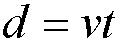So, for example, if an object moved with a constant velocity of 5 m/s for 3 s, then it would be displaced 15 m. Here is an example of that calculation: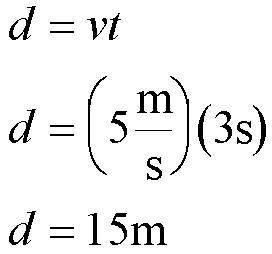The above equation could be rearranged using algebra into other forms. Here are all of its forms: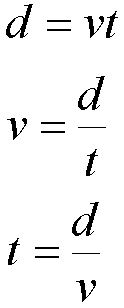Often the velocity of an object is not constant. It can change as time passes. When this happens, you can calculate an average velocity for the object. You need to know the total displacement and the amount of time that passes during that total displacement. Using those values, when we divide the displacement by time, we get a value that is known as the average velocity. Here is an equation for average velocity: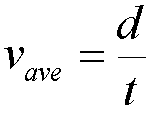In the above equation d is the displacement from the object's starting position to its ending position, and t is the time over which the displacement occurred. Knowing d and t, we can calculate an average velocity. However, we can not claim to know exactly what the velocity was at a certain instant in time, just the average velocity over the whole time period.

Custom Search